Request a Free Counselling Session from our Expert Mentor# NCERT Solution for Class 10th Maths Chapter 10 Circles

In this chapter, you will learn about the properties of circles and their various parts, such as chords, arcs, tangents, and sectors. You will also study the relationships between the angles formed by these parts and their corresponding lengths.
Swastik Classes provides detailed and step-by-step solutions to all the exercises and examples in the NCERT textbook. Our solutions are designed to help you understand the concepts clearly and concisely. We also provide additional practice questions to help you reinforce your understanding of the chapter.
We aim to help you achieve a deep understanding of the subject matter and develop your problem-solving skills. We believe that a strong foundation in mathematics is essential for academic success and future career opportunities. So, let’s dive into the world of circles and explore the fascinating properties they possess.

## Answers of Math NCERT solutions for Class 10th Maths Chapter 10 Circles

Unit 10

Circles

Exercise 10.1, 10.2 Solutions

Exercise 10.1

Q1 :

How many tangents can a circle have?

A circle can have infinite tangents.

Q2 :

Fill in the blanks:

1.         A tangent to a circle intersects it in    points.
2.         A line intersecting a circle in two points is called a    .
3.     A circle can have parallel tangents at the most.
4.     The common point of a tangent to a circle and the circle is called    .

1.         One
2.     Secant
3. Two
4. Point of contact

Q3 :

A tangent PQ at a point P of a circle of radius 5 cm meets a line through the centre O at a point Q so that OQ

= 12 cm. Length PQ is :

(A) 12 cm.         (B) 13 cm         (C) 8.5 cm         (D)

We know that the line drawn from the centre of the circle to the tangent is perpendicular to the tangent.

OPPQ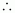By applying Pythagoras theorem in ΔOPQ,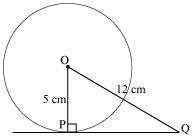∴ OP2 + PQ2 = OQ2 52 + PQ2 =122

PQ2 =144 – 25

PQ =

Hence, the correct answer is (D).

Q4 :

Draw a circle and two lines parallel to a given line such that one is a tangent and the other, a secant to the circle.

Answer :It can be observed that AB and CD are two parallel lines. Line AB is intersecting the circle at exactly two points, P and Q. Therefore, line AB is the secant of this circle. Since line CD is intersecting the circle at exactly one point, R, line CD is the tangent to the circle.

Exercise 10.2

Q1 :

From a point Q, the length of the tangent to a circle is 24 cm and the distance of Q from the centre is 25 cm.

The radius of the circle is

(A)        7 cm         (B)     12 cm     (C)     15 cm     (D)     24.5 cm

Answer :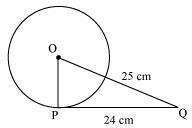Let O be the centre of the circle. Given that,

OQ = 25cm and PQ = 24 cm

As the radius is perpendicular to the tangent at the point of contact, Therefore, OP ⊥ PQ

Applying Pythagoras theorem in ΔOPQ, we obtain OP2 + PQ2 = OQ2

OP = 7

Therefore, the radius of the circle is 7 cm. Hence, (A) is correct.

Q2 :

In the given figure, if TP and TQ are the two tangents to a circle with centre O so that ∠POQ = 110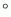, then

∠PTQ is equal to

(A) 60°              (B) 70°        (C) 80°          (D) 90°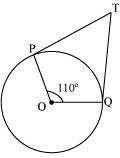It is given that TP and TQ are tangents.

Therefore, radius drawn to these tangents will be perpendicular to the tangents. Thus, OP ⊥ TP and OQ ⊥ TQ

∠OPT = 90º

∠OQT = 90º

Sum of all interior angles = 360°

∠OPT + ∠POQ +∠OQT + ∠PTQ = 360°

⇒ 90º  + 110 º + 90º  + ∠PTQ = 360°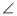PTQ = 70°

Hence, alternative (B) is correct.

Q3 :

If tangents PA and PB from a point P to a circle with centre O are inclined to each other an angle of 80°, then ∠POA is equal to

(A) 50°            (B) 60°        (C) 70°         (D) 80°

It is given that PA and PB are tangents.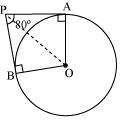Therefore, the radius drawn to these tangents will be perpendicular to the tangents. Thus, OA ⊥ PA and OB ⊥ PB

∠OBP = 90 º

∠OAP = 90 º In AOBP,

Sum of all interior angles = 360°

∠OAP + ∠APB +∠PBO + ∠BOA = 360°

90°  + 80° + 90° + ∠BOA = 360°

∠BOA = 100°

In ΔOPB and ΔOPA,

AP = BP (Tangents from a point)

OA = OB (Radii of the circle)

OP = OP (Common side)

Therefore, ΔOPB ~ ΔOPA (SSS congruence criterion) A ↔ B, P ↔ P, O ↔ O

And thus, ∠POB = ∠POA

Hence, alternative (A) is correct.

Q4 :

Prove that the tangents drawn at the ends of a diameter of a circle are parallel.

Answer :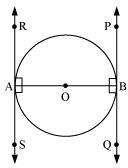Let AB be a diameter of the circle. Two tangents PQ and RS are drawn at points A and B respectively. Radius drawn to these tangents will be perpendicular to the tangents.

Thus, OA ⊥ RS and OB ⊥ PQ

∠ OAR = 90º

∠ OAS = 90º

∠ OBP = 90º

∠ OBQ = 90º

It can be observed that

∠ OAR = ∠ OBQ (Alternate interior angles)

∠ OAS = ∠ OBP (Alternate interior angles)

Since alternate interior angles are equal, lines PQ and RS will be parallel.

Q5 :

Prove that the perpendicular at the point of contact to the tangent to a circle passes through the centre.

Let us consider a circle with centre O. Let AB be a tangent which touches the circle at P.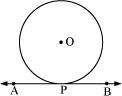We have to prove that the line perpendicular to AB at P passes through centre O. We shall prove this by contradiction method.

Let us assume that the perpendicular to AB at P does not pass through centre O. Let it pass through another point O’. Join OP and O’P.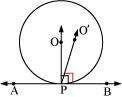As perpendicular to AB at P passes through O’, therefore,

∠ O’PB = 90°     … (1)

O is the centre of the circle and P is the point of contact. We know the line joining the centre and the point of contact to the tangent of the circle are perpendicular to each other.

∴ ∠ OPB = 90°     .. (2)

Comparing equations (1) and (2), we obtain

∠ O’PB = ∠ OPB     … (3)

From the figure, it can be observed that,

∠ O’PB < ∠ OPB     … (4)

Therefore, ∠ O’PB = ∠ OPB is not possible. It is only possible, when the line O’P coincides with OP. Therefore, the perpendicular to AB through P passes through centre O.

Q6 :

The length of a tangent from a point A at distance 5 cm from the centre of the circle is 4 cm. Find the radius of the circle.

Answer :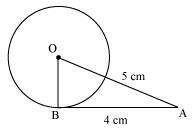Let us consider a circle centered at point O.

AB is a tangent drawn on this circle from point A. Given that,

OA = 5cm and AB = 4 cm In ΔABO,

OB ⊥ AB (Radius ⊥ tangent at the point of contact) Applying Pythagoras theorem in ΔABO, we obtain AB2 + BO2 = OA2

42 + BO= 52

16 + BO2 = 25

BO2 = 9

BO = 3

Hence, the radius of the circle is 3 cm.

*Q7 :

Two concentric circles are of radii 5 cm and 3 cm. Find the length of the chord of the larger circle which touches the smaller circle.

Let the two concentric circles be centered at point O. And let PQ be the chord of the larger circle which touches the smaller circle at point A. Therefore, PQ is tangent to the smaller circle.

OA ⊥ PQ (As OA is the radius of the circle) Applying Pythagoras theorem in ΔOAP, we obtain OA2 + AP2 = OP2

32 + AP2 = 52

9 + AP2 = 25

AP2 = 16

AP = 4

In ΔOPQ,

Since OA ⊥ PQ,

AP = AQ (Perpendicular from the center of the circle bisects the chord) PQ = 2AP = 2 × 4 = 8

∴ Therefore, the length of the chord of the larger circle is 8 cm.

*Q8 :

A quadrilateral ABCD is drawn to circumscribe a circle (see given figure) Prove that AB + CD = AD + BC

It can be observed that

DR = DS (Tangents on the circle from point D)         … (1)

CR = CQ (Tangents on the circle from point C)         … (2)

BP = BQ (Tangents on the circle from point B)         … (3)

AP = AS (Tangents on the circle from point A)         … (4)

Adding all these equations, we obtain

DR + CR + BP + AP = DS + CQ + BQ + AS

(DR + CR) + (BP + AP) = (DS + AS) + (CQ + BQ) CD + AB = AD + BC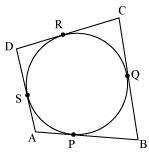*Q9 :

In the given figure, XY and X’Y’ are two parallel tangents to a circle with centre O and another tangent AB with point of contact C intersecting XY at A and X’Y’ at B. Prove that ∠AOB=90.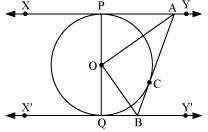Let us join point O to C.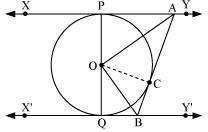In ΔOPA and ΔOCA,

OP = OC (Radii of the same circle)

AP = AC (Tangents from point A)

AO = AO (Common side)

ΔOPA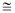ΔOCA (SSS congruence criterion)

Therefore, P ↔ C, A ↔ A, O ↔ O

∠POA = ∠COA         … (i)

Similarly, ΔOQBΔOCB

∠QOB = ∠COB         … (ii)

Since POQ is a diameter of the circle, it is a straight line. Therefore, ∠POA + ∠COA + ∠COB + ∠QOB = 180 º From equations (i) and (ii), it can be observed that 2∠COA + 2 ∠COB = 180 º

∠COA + ∠COB = 90 º

∠AOB = 90°

Q10 :

Prove that the angle between the two tangents drawn from an external point to a circle is supplementary to the angle subtended by the line-segment joining the points of contact at the centre.

Answer :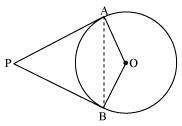Let us consider a circle centered at point O. Let P be an external point from which two tangents PA and PB are drawn to the circle which are touching the circle at point A and B respectively and AB is the line segment, joining point of contacts A and B together such that it subtends ∠ AOB at center O of the circle.

It can be observed that

OA (radius) ⊥ PA (tangent) Therefore, ∠ OAP = 90°

Similarly, OB (radius) ⊥ PB (tangent)

∠ OBP = 90°

Sum of all interior angles = 360º

∠ OAP +∠ APB+∠ PBO +∠ BOA = 360º 90º + ∠ APB + 90º + ∠ BOA = 360º

∠ APB + ∠ BOA = 180º

Hence, it can be observed that the angle between the two tangents drawn from an external point to a circle is supplementary to the angle subtended by the line-segment joining the points of contact at the centre.

Q11 :

Prove that the parallelogram circumscribing a circle is a rhombus.

Since ABCD is a parallelogram, AB = CD     …(1)

BC = AD                        …(2)It can be observed that

DR = DS (Tangents on the circle from point D)

CR = CQ (Tangents on the circle from point C)

BP = BQ (Tangents on the circle from point B)

AP = AS (Tangents on the circle from point A)

Adding all these equations, we obtain

DR + CR + BP + AP = DS + CQ + BQ + AS

(DR + CR) + (BP + AP) = (DS + AS) + (CQ + BQ) CD + AB = AD + BC

On putting the values of equations (1) and (2) in this equation, we obtain 2AB = 2BC

AB = BC                         …(3)

Comparing equations (1), (2), and (3), we obtain AB = BC = CD = DA

Hence, ABCD is a rhombus.

*Q12 :

A triangle ABC is drawn to circumscribe a circle of radius 4 cm such that the segments BD and DC into which BC is divided by the point of contact D are of lengths 8 cm and 6 cm respectively (see given figure). Find the sides AB and AC.

Answer :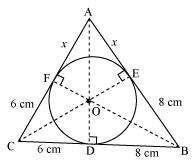Let the given circle touch the sides AB and AC of the triangle at point E and F respectively and the length of the line segment AF be x.

In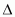ABC,

CF = CD = 6cm (Tangents on the circle from point C)

BE = BD = 8cm (Tangents on the circle from point B)

AE = AF = x (Tangents on the circle from point A)

AB = AE + EB = x + 8

BC = BD + DC = 8 + 6 = 14

CA = CF + FA = 6 + x

2s = AB + BC + CA

= x + 8 + 14 + 6 + x

= 28 + 2x

s = 14 + x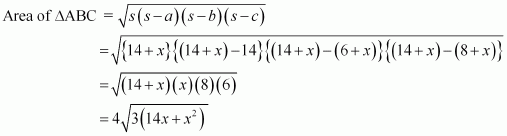Area of

Area of

Area of

Area of ΔABC = Area of ΔOBC + Area of ΔOCA + Area of ΔOAB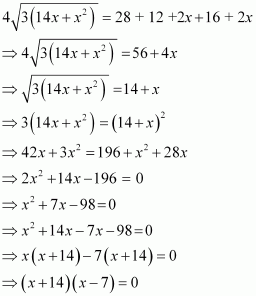Either x+14 = 0 or x – 7 =0 Therefore, x = – 14and 7

However, x = – 14 is not possible as the length of the sides will be negative. Therefore, x = 7

Hence, AB = x + 8 = 7 + 8 = 15 cm

CA = 6 + x = 6 + 7 = 13 cm

*Q13 :

Prove that opposite sides of a quadrilateral circumscribing a circle subtend supplementary angles at the centre of the circle.

Let ABCD be a quadrilateral circumscribing a circle centered at O such that it touches the circle at point P, Q, R, S. Let us join the vertices of the quadrilateral ABCD to the center of the circle.

Consider ΔOAP and ΔOAS,

AP = AS (Tangents from the same point) OP = OS (Radii of the same circle)

OA = OA (Common side)

ΔOAPΔOAS (SSS congruence criterion)

Therefore, ΔOAPΔOAS and thus, ∠ POA = ∠ AOS

∠ 1 = ∠ 8

Similarly,

∠ 2 = ∠ 3

∠ 4 = ∠ 5

∠ 6 = ∠ 7

∠ 1 + ∠ 2 + ∠ 3 + ∠ 4 + ∠ 5 + ∠ 6 + ∠ 7 + ∠ 8 = 360º

(∠ 1 + ∠ 8) + (∠ 2 + ∠ 3) + (∠ 4 + ∠ 5) + (∠ 6 + ∠ 7) = 360º

2∠ 1 + 2∠ 2 + 2∠ 5 + 2∠ 6 = 360º

2(∠ 1 + ∠ 2) + 2(∠ 5 + ∠ 6) = 360º

(∠ 1 + ∠ 2) + (∠ 5 + ∠ 6) = 180º

∠ AOB + ∠ COD = 180º

Similarly, we can prove that ∠ BOC + ∠ DOA = 180º

Hence, opposite sides of a quadrilateral circumscribing a circle subtend supplementary angles at the centre of the circle.

## NCERT Solutions for Class 10 Maths Chapter 10 Circles

This chapter comes under Unit 6 and has a weightage of 6 marks in the board examination. There will be one mark MCQ question, 2 marks reasoning questions and 3 marks short answer questions. This chapter has fundamental concepts that lay the foundation for your future studies.

The chapter Circle is included in Unit 4 Geometry of CBSE syllabus 2019-20. This unit has a weightage of 22 marks allotted in class 10 board examination. Unit 4 will have 4 MCQs carrying 4 marks, 2 Short answer questions carrying 6 marks and two long answer questions carrying 12 marks.

### Sub-topics of Class 10 Chapter 10 Circles

• Introduction to Circles
• Tangent to a circle
• Number of Tangents from a point on a circle
• Summary of the Whole Chapter

### List of Exercise from Class 10 Maths Chapter 10 Circles

Exercise 10.1– 4 Questions which includes 1 short answer questions, 1 fill in the blanks question and 2 long answer questions

Exercise 10.2– 13 Questions which includes 10 long answer questions, 4 descriptive type questions and 2 short answer questions

The Solutions for NCERT class 10 will guide you to understand the concepts involved in circles. You can refer this for better understanding of the concept. This Solution will also aid you to score good marks in the examination.

Class 10 Maths Chapter 10 deals with the existence of the tangents to a circle and some of the properties of circle. Students are introduced to some complex terms such as tangents, tangents to a circle, number of tangents from a point on the circle. This chapter seems very interesting due to the diagrams and involvement of geometrical calculations.

This NCERT Solution Class 10 Maths Chapter 10 has some tricky concept question on circles which will help you to clear all your doubts when you study. Students are recommended to study these solutions to know alternative calculation methods.

### Key Features of NCERT Solutions for Class 10 Maths Chapter 10 Circles

• Provide answers to all the exercise questions in the NCERT class 10 Maths textbook.
• Create a practise of tricky questions which will clear your understanding of the topics.
• Covers entire syllabus and possible types of questions to be asked in the examination.
• Helps you to practise important drawings.
• Aids you in memorizing important formulas and calculation methods.

Conclusion

The NCERT Solutions for Class 10 Maths Chapter 10 – Circles, provided by Swastik Classes, offer comprehensive and well-structured solutions to all the exercises and examples in the NCERT textbook. The chapter focuses on the properties of circles and their different parts, such as chords, arcs, tangents, and sectors, along with the relationships between the angles formed by these parts and their corresponding lengths.
Our solutions are designed to help students understand the concepts in a clear and concise manner, which can assist in building a strong foundation in mathematics. We also provide additional practice questions to help students strengthen their problem-solving skills and reinforce their understanding of the chapter.
At Swastik Classes, our goal is to help students achieve their academic and career aspirations by providing them with quality education and guidance. We hope that our NCERT Solutions for Class 10 Maths Chapter 10 – Circles will prove to be helpful in this regard.

## Frequently Asked Questions on NCERT Solutions for Class 10 Maths Chapter 10

### What are the key features of NCERT Solutions for Class 10 Maths Chapter 10?

NCERT Solutions for Class 10 Maths Chapter 10 provides solutions to all the exercise questions in the NCERT class 10 Maths Chapter 10. Also makes a practise of tricky questions which will clear your understanding of the topics.

### What are the main topics that are covered in NCERT Solutions for Class 10 Maths Chapter 10?

The main topics that are covered in NCERT Solutions for Class 10 Maths Chapter 10 are introduction to circles, tangent to a circle, number of tangents from a point on a circle and summary of the whole chapter.

### Is it necessary to learn all the questions provided in NCERT Solutions for Class 10 Maths Chapter 10?

Yes, you must learn all the questions provided in NCERT Solutions for Class 10 Maths Chapter 10. Because these questions may appear in board exams as well in class tests. By learning these questions students will be ready for their upcoming exams.